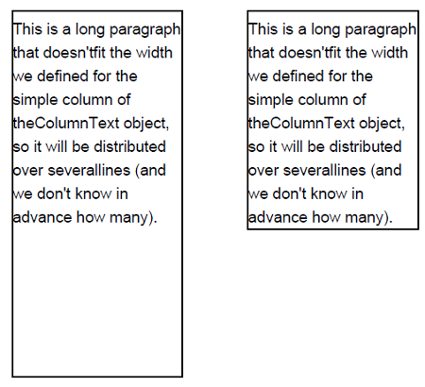iText pdf library

# How to draw a rectangle around multiline text?

I am trying to draw a rectangle around multi-line text in iText. I use this code to draw the text:

```ColumnText ct = new ColumnText(cb);
Phrase phrase = new Phrase("Some String\nOther string etc...\n test");
ct.setSimpleColumn(myText......);
ct.go();```
I know how to draw a rectangle, but I am not able to draw a rectangle outlining this text.

Posted on StackOverflow on Mar 13, 2012 by user2439522

It sounds as if you are missing only a single piece of the puzzle to meet your requirement. That piece is called `getYLine()`.

Please take a look at the DrawRectangleAroundText example. This example draws the same paragraph twice. The first time, it adds a rectangle that probably looks like the solution you already have. The second time, it adds a rectangle the way you want it to look:Screen shot

The first time, we add the text like this:

``````ColumnText ct = new ColumnText(cb);
ct.setSimpleColumn(120f, 500f, 250f, 780f);
Paragraph p = new Paragraph("This is a long paragraph that doesn't"
+ "fit the width we defined for the simple column of the"
+ "ColumnText object, so it will be distributed over several"
+ "lines (and we don't know in advance how many).");
ct.go();
``````

You define your column using the coordinates:

``````llx = 120;
lly = 500;
urx = 250;
ury = 780;
``````

This is a rectangle with lower left corner (120, 500), a width of 130 and a height of 380. Hence you draw a rectangle like this:

``````cb.rectangle(120, 500, 130, 280);
cb.stroke();
``````

Unfortunately, that rectangle is too big.

Now let's add the text once more at slightly different coordinates:

``````ct = new ColumnText(cb);
ct.setSimpleColumn(300f, 500f, 430f, 780f);
ct.go();
``````

Instead of using (300, 500) as lower left corner for the rectangle, we ask the `ct` object for its current Y position using the `getYLine()` method:

``````float endPos = ct.getYLine() - 5;
``````

As you can see, I subtract 5 user units, otherwise the bottom line of my rectangle will coincide with the baseline of the final line of text and that doesn't look very nice. Now I can use the `endPos` value to draw my rectangle like this:

``````cb.rectangle(300, endPos, 130, 780 - endPos);
cb.stroke();
``````

Contact

## Still have questions?

We're happy to answer your questions. Reach out to us and we'll get back to you shortly.